ICSE Class 9 Logarithms Solution New Pattern

## ICSE Class 9 Logarithms Solution New Pattern By Clarify Knowledge

ICSE Class 9 Logarithms Solution New Pattern 2022

## Chapter 8 - Logarithms Exercise Ex. 8(A)

Question 1

Express each of the following in logarithmic form:

(i) 53 = 125

(ii) 3-2 =(iii) 10-3 = 0.001

(iv) Solution 1

(i)

(ii)

(iii)

(iv)

Question 2

Express each of the following in exponential form:

(i) logg 0.125 = -1

(ii) log100.01 = -2

(iii) logaA = x

(iv) log101 = 0Solution 2

(i)

(ii)

(iii)

(iv)

Question 3

Solve for x: log10 x = -2.Solution 3

Question 4

Find the logarithm of:

(i) 100 to the base 10

(ii) 0.1 to the base 10

(iii) 0.001 to the base 10

(iv) 32 to the base 4

(v) 0.125 to the base 2

(vi)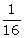to the base 4

(vii) 27 to the base 9

(viii)to the base 27Solution 4

(i)

(ii)

(iii)

(iv)

(v)

(vi)

(vii)

(viii)

Question 5

State, true or false:

(i) If log10 x = a, then 10x = a.

(ii) If xy = z, then y = logzx.

(iii) log2 8 = 3 and log8 = 2 =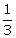.Solution 5

(i)

(ii)

(iii)

Question 6

Find x, if:

(i) log3 x = 0

(ii) logx 2 = -1

(iii) log9243 = x

(iv) log5 (x - 7) = 1

(v) log432 = x - 4

(vi) log7 (2x2 - 1) = 2Solution 6

(i)

(ii)

(iii)

(iv)

(v)

(vi)

Question 7

Evaluate:

(i) log10 0.01

(ii) log2 (1 ÷ 8)

(iii) log5 1

(iv) log5 125

(v) log16 8

(vi) log0.5 16Solution 7

(i)

(ii)

(iii)

(iv)

(v)

(vi)

Question 8

If loga m = n, express an - 1 in terms in terms of a and m.Solution 8

Question 9

Solution 9

Question 10

Solution 10

Question 11

Solution 11

Question 12

If log (x2 - 21) = 2, show that x = ± 11.Solution 12

## Chapter 8 - Logarithms Exercise Ex. 8(B)

Question 1

Express in terms of log 2 and log 3:

(i) log 36 (ii) log 144 (iii) log 4.5

(iv) log- log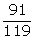(v) loglog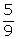+ logSolution 1

(i)

(ii)

(iii)

(iv)

(v)

Question 2

Express each of the following in a form free from logarithm:

(i) 2 log x - log y = 1

(ii) 2 log x + 3 log y = log a

(iii) a log x - b log y = 2 log 3Solution 2

(i)

(ii)

(iii)

Question 3

Evaluate each of the following without using tables:

(i) log 5 + log 8 - 2 log 2

(ii) log108 + log1025 + 2 log103 - log1018

(iii) log 4 +log 125 -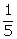log 32Solution 3

(i)(ii)(iii)Question 4

Prove that:

Solution 4

Question 5

Find x, if:

x - log 48 + 3 log 2 =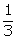log 125 - log 3.Solution 5

Question 6

Express log102 + 1 in the form of log10x.Solution 6

Question 7

Solve for x:

(i) log10 (x - 10) = 1

(ii) log (x2 - 21) = 2

(iii) log (x - 2) + log (x + 2) = log 5

(iv) log (x + 5) + log (x - 5)

= 4 log 2 + 2 log 3Solution 7

(i)

(ii)

(iii)

(iv)

Question 8

Solve for x:

(i)(ii)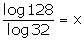(iii)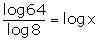(iv)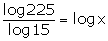Solution 8

(i)

(ii)

(iii)

(iv)

Question 9

Given log x = m + n and log y = m - n, express the value oflog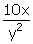in terms of m and n.Solution 9

Question 10

State, true or false:

(i) log 1log 1000 = 0

(ii)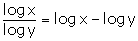(iii) If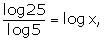then x = 2

(iv) log xlog y = log x + log ySolution 10

(i)

(ii)

(iii)

(iv)

Question 11

If log102 = a and log103 = b; express each of the following in terms of 'a' and 'b':

(i) log 12(ii) log 2.25(iii) log(iv) log 5.4(v) log 60(iv) log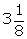Solution 11

(i)

(ii)

(iii)

(iv)

(v)

(vi)

Question 12

If log 2 = 0.3010 and log 3 = 0.4771; find the value of:

(i) log 12(ii) log 1.2(iii) log 3.6

(iv) log 15(v) log 25(vi)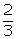log 8Solution 12

(i)

(ii)

(iii)

(iv)

(v)

(vi)

Question 13

Given 2 log10 x + 1 = log10 250, find :

(i) x(ii) log10 2xSolution 13

(i)

(ii)

Question 14

Solution 14

Question 15

Solution 15

Question 16

Solution 16

## Chapter 8 - Logarithms Exercise Ex. 8(C)

Question 1

If log10 8 = 0.90; find the value of:

(i) log10 4(ii) log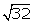(iii) log 0.125Solution 1

(i)

(ii)

(iii)

Question 2

If log 27 = 1.431, find the value of :

(i) log 9(ii) log 300Solution 2

(i)

(ii)

Question 3

If log10 a = b, find 103b - 2 in terms of a.Solution 3

Question 4

If log5 x = y, find 52y+ 3 in terms of x.Solution 4

Question 5

Given: log3 m = x and log3 n = y.

(i) Express 32x - 3 in terms of m.

(ii) Write down 31 - 2y + 3x in terms of m and n.

(iii) If 2 log3 A = 5x - 3y; find A in terms of m and n.Solution 5

(i)

(ii)

(iii)

Question 6

Simplify:

(i) log (a)3 - log a

(ii) log (a)3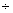log aSolution 6

(i)

(ii)

Question 7

If log (a + b) = log a + log b, find a in terms of b.Solution 7

Question 8

Prove that:

(i) (log a)2 - (log b)2 = log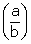. Log (ab)

(ii) If a log b + b log a - 1 = 0, then ba. ab = 10Solution 8

(i)

(ii)

Question 9

(i) If log (a + 1) = log (4a - 3) - log 3; find a.

(ii) If 2 log y - log x - 3 = 0, express x in terms of y.

(iii) Prove that: log10 125 = 3(1 - log102).Solution 9

(i)

(ii)

(iii)

Question 10

Solution 10

Question 11

Solution 11

## Chapter 8 - Logarithms Exercise Ex. 8(D)

Question 1

If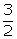log a +log b - 1 = 0, find the value of a9.b4.Solution 1

Question 2

If x = 1 + log 2 - log 5, y = 2 log3 and z = log a - log 5; find the value of a if x + y = 2z.Solution 2

Question 3

If x = log 0.6; y = log 1.25 and z = log 3 - 2 log 2, find the values of:

(i) x+y- z        (ii) 5x + y - zSolution 3

(i)

(ii)

Question 4

If a2 = log x, b3 = log y and 3a2 - 2b3 = 6 log z, express y in terms of x and z.Solution 4

Question 5

If log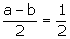(log a + log b), show that: a+ b2 = 6ab.Solution 5

Question 6

If a2 + b2 = 23ab, show that:

log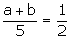(log a + log b).Solution 6

Question 7

If m = log 20 and n = log 25, find the value of x, so that: 2 log (x - 4) = 2 m - n.Solution 7

Question 8

Solve for x and y ; if x > 0 and y > 0;log xy = log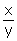+ 2 log 2 = 2.Solution 8

Question 9

Find x, if:

(i) logx 625 = -4

(ii) logx (5x - 6) = 2Solution 9

(i)

(ii)

Question 10

Solution 10

Question 11

Solution 11

Question 12

Solution 12

Question 13

Given log10x = 2a and log10y = .

(i) Write 10a in terms of x.

(ii) Write 102b + 1 in terms of y.

(iii) If , express P in terms of x and y.Solution 13

Question 14

Solve:

log5(x + 1) - 1 = 1 + log5(x - 1).Solution 14

Question 15

Solve for x, if:

Solution 15

Question 16

Solution 16

Question 17

Solution 17

Question 18

Solution 18

Question 19

Solution 19

Question 20

Solution 20

Question 21

Solution 21

Question 22

Solution 22

error: Content is protected !!